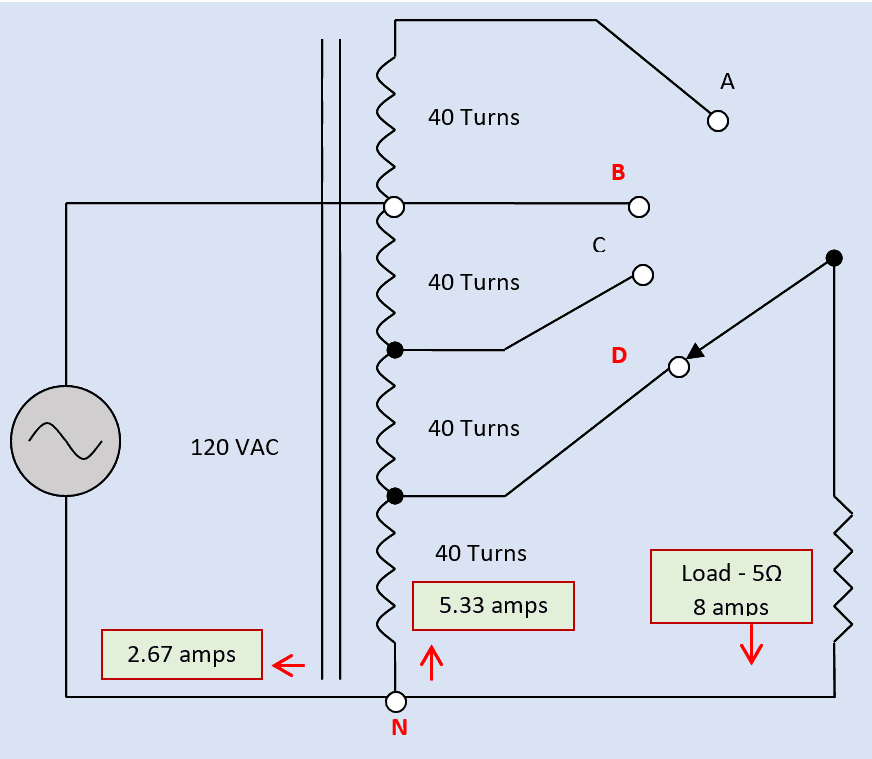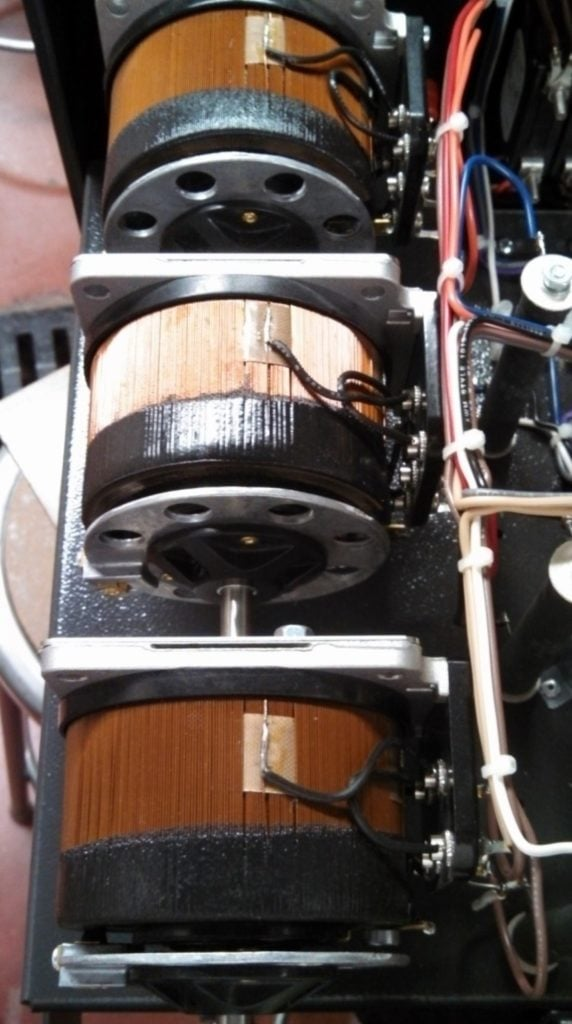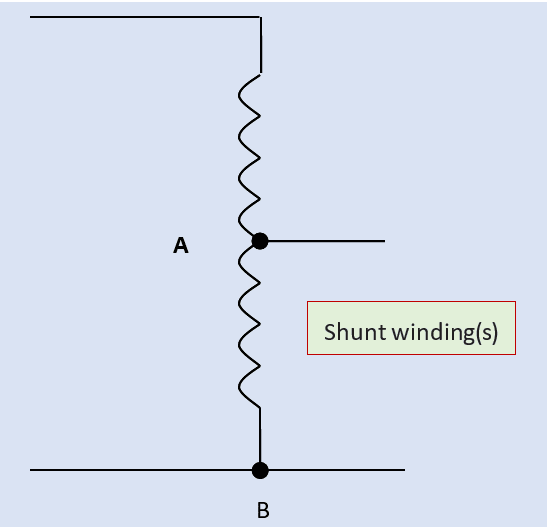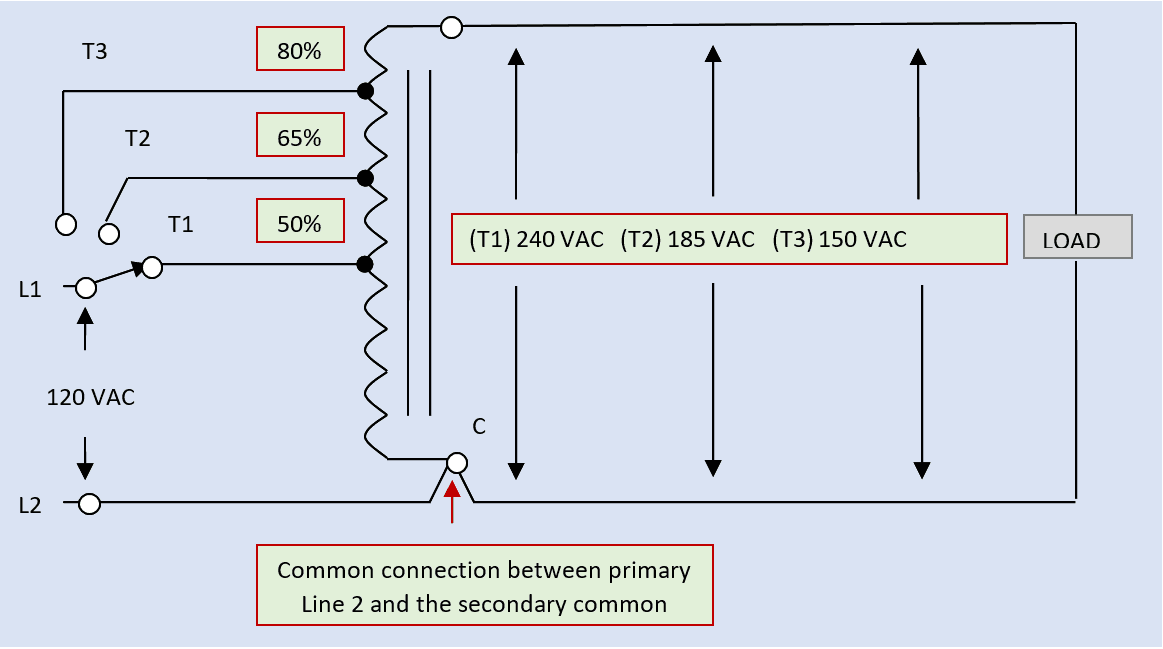Want create site? Find Free WordPress Themes and plugins.

An autotransformer is nothing more than a reactor coil (single winding), but when tapped along a portion of the single winding, it will function as a single-phase single-winding AC transformer. The same winding, as shown in Figure 1, or at least part of it, is used for both the primary and secondary windings. The other end of the winding marked N in the drawing is the common connection of both the secondary and primary circuits. Autotransformers are often used because of their lower installation costs (lower manufacturing costs) as compared to conventional (isolation) transformers.

The tapped single-winding auto-transformer will obey all the laws of transformation (voltage, current, and circuit impedance) except isolation. The common connection N in Figure 1 makes a physical and electrical connection between the primary and secondary circuits.

## Autotransformer Working Principle

Four taps, labeled as A, B, C, and D settings on the selector switch, are shown for the secondary circuit of the autotransformer in Figure 1. A voltage of 120 VAC (Volts AC) is applied to the primary winding, which is connected between points (taps) B and N on the winding. As indicated in the drawing, there are a total of 40 turns between each tap on the autotransformer. Totaling the turns of wire between points B and N, the 120-volt supply is being applied to 120 turns. According to the selected position of the selector switch, each turn will yield one volt. In other words, at the selected switch position D, as indicated in the figure, 40 volts is being applied to the connected load.

By formula:

VP × NS = VS × NP

Rearranging:

VS × NP = VP × NS

Solving for VS:

VS = (VP × NS) ÷ NP

VS = VP × (NS ÷ NP)

VS = 120 volts × (40 turns ÷ 120 turns) = 40 volts

If the load connected to the secondary has a rated impedance of 5 ohms, the secondary current should equal 8 amps. By Ohm’s law:

A load = 40 volts ÷ 5 W = 8 amps

The primary current can be projected from this secondary load current using the inverse of the voltage ratio.

By formula:

VP × AP = VS × AS

Solving for AP

AP = (VS × AS) ÷ VP

Rearranging

AP = (VS ÷ VP) × AS

A primary = (40 volts ÷ 120 volts) × 8 amps = 2.67 amps

The amount of power input and output for the autotransformer must be the same.

VP × AP = VS × AS (Between terminals D and N)

120 volts × 2.67 amps = 40 volts × 8 amps

320.4 watts = 320 watts (Rounding Error)

The amount of current flowing through the 40-turn coil that is common to both the primary and secondary circuits can be a bit confusing. In terms of nodal analysis, Kirchhoff’s current law states that all the current flowing into a node (common point), must flow out of the node. From the load, 8 amps flow into the common node on the autotransformer (marked N in Figure 1). From the common node, 2.67 amps flow out to the power supply. The difference between these two currents equals the amount of current flowing through the winding:

8 amps – 2.67 amps = 5.33 ampsFigure 1. Diagram of a single-winding tapped autotransformer circuit

At the other end, which is the D tap node on the autotransformer winding: 2.67 amps flow from the power supply from tap B down through the upper part of the winding; 5.33 amps flow up from the lower part of the winding, and 8 amps (their sum) flow out to the connected load.

Like the isolation transformer, the autotransformer can be either a step-up or step-down transformer. Tap-point A in Figure 1 puts an additional 40 turns of wire in the secondary as compared to the primary. At 1-volt per turn (established by the tap point of the power supply), if the selector switch was rotated to the A setting, 160 volts would be applied to the connected load.

If the rotary switch shown in Figure 1 is replaced with a sliding tap that makes contact directly to the transformer winding, the turns ratio can be adjusted continuously. This type of autotransformer, which is technically a rheostat, is commonly referred to as a Variac.

Instead of a straight section of the iron core, the iron core in the continuous-tap autotransformer is normally shaped into a cylinder and the continuous insulated wire in the winding of the autotransformer is wrapped tightly — one turn after the other — around the iron-core cylinder (Figure 2). Once the winding (wrapping) is completed, a grinder is used to remove the insulation off of the crown of the wire in the winding on one side of the cylindrical iron core. Adding the wiper from the one-turn rotary switch allows any voltage, within the rating of the primary supply voltage, to be “picked off” the secondary continuous coil.Figure 2. A rheostat is an autotransformer manufactured with a sliding tap

Again, the one disadvantage to using the autotransformer is the lack of isolation between the primary supply and secondary load circuit — the load is connected to one side of the power line on one end of the autotransformer winding. This can cause problems with certain types of equipment and must be a consideration when designing an AC electrical-power circuit or distribution system.

Remember the input to any transformer, which is the primary of the transformer, can be either the higher- or lower-voltage winding of the transformer. [See Figure 3]Figure 3. Autotransformer

If the autotransformer will be used in a step-down voltage configuration, the input (primary) would be connected across the full winding, and the secondary lower-voltage output would be taken from reference-points A and B.

If the autotransformer will be used in a step-up voltage configuration, the input (primary) would be connected across the tap reference-points A and B, and the secondary higher-voltage output would be taken across the full winding.Figure 4. The values of full-winding voltage from a step-up autotransformer are determined by dividing the primary tap voltage rating by the tap-percentage values

## Autotransformer Use

Another common use of an autotransformer is the adjustment or compensation for voltage drop: Compensation is required when voltage drop, due to an overall length of the circuit conductors and the magnitude of the full-load current, reduces the supply voltage below the rated voltage of an electric motor or a resistive load. The autotransformer, as shown in Figure 4, is wired in a step-up configuration: The lower voltage is connected between one of the taps and the common winding terminal. The higher voltage is derived from the full-winding connections. The percentage-value of the tap is divided into the primary (lower) voltage rating to determine the secondary (higher) voltage rating. The 80% supply tap will boost the load voltage up to 125% of the supply voltage. The 50% supply tap will boost the load voltage up to 200% of the supply voltage.

Did you find apk for android? You can find new Free Android Games and apps.